Section Data Created Edited

# Common

## Function

• Enter section properties for line elements (Truss, Tension-only, Compression-only, Cable, Gap, Hook, Beam Element).

## Call

From main menu, select [Properties] tab > [Section] group >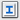[Section Properties] > [Common]

## Input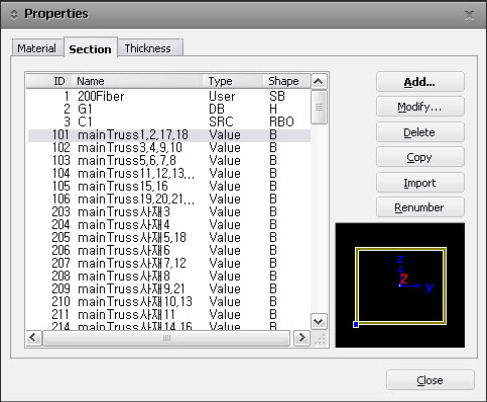Properties(Section) dialog box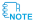The section number can be entered up to a maximum of six digits. 

### Modify...

Modify the existing section(s)

### Delete

Delete the existing section(s)

### Copy

Copy the existing section data. Select the section to be copied from the list and click the button.

### Import

Load the section data from the existing fn.mcb file where section data is already inputted.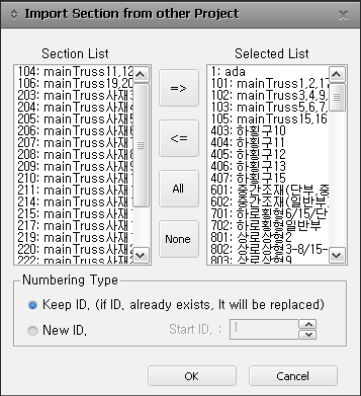Section List

The section data inputted in the existing fn.MCB file is displayed.

Selected List

Select the section data you want to import and register it in the list.When selecting fn.MCB, all the existing section data inputted in fn.MCB is registered in the Selected List.

Numbering Type

Specify the import method for the section number.

Keep ID

Import the section numbers from the existing fn.MCB file using the same numbering scheme.

New ID

Assign new numbers to the section data being imported.

### Renumbering

Change the numbers of the existing section data that has already been entered.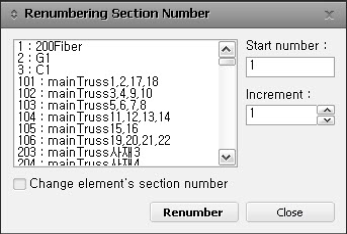Start number

Enter the starting number for the section numbers to be changed.

Increment number

Enter the increment value for the section property data numbers.

Change element's material number

Change the section numbers of the elements. By using this function, the defined section numbers will be modified. If this function is not used, the selected existing section numbers will be converted to an "Undefined" state without a section name defined, and additionally, a user-specified material number will be created for the elements without any section assignment.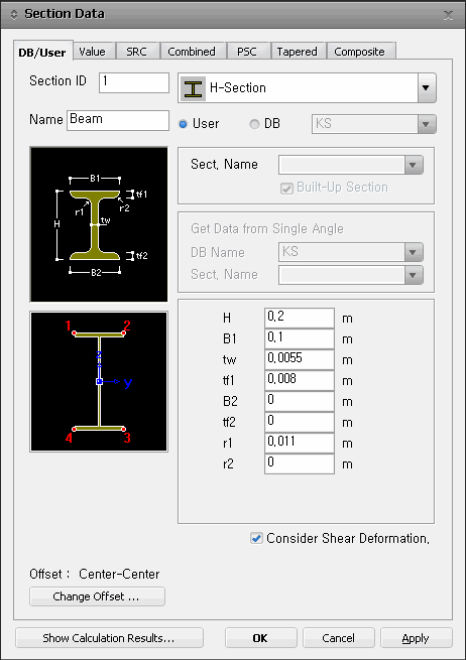Section data definition dialog box

### Section ID

The section number will be automatically set to the final section number entered plus one.

### Name

The section name will be automatically assigned as "Sect. Name" if no input content is provided.

### Consider Shear Deformation

Select whether to consider shear deformation. This option is applied during structural analysis and does not affect the effective shear area (Asy, Asz) data that appears when clicking the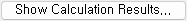button.

### Offset

Specify the position of the centroid of the section.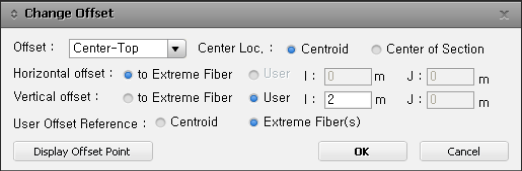Change Offset dialog box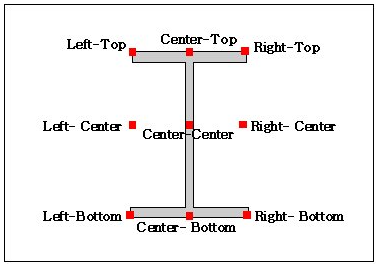Offset location

Center Loc. : Choose the position of the center between the centroid and the center of section dimensions.

Horizontal Offset : Specify the lateral offset position of the section. If "to Extreme Fiber" is selected, the position specified in "Offset" will be reflected as shown in the illustration above. If you want to specify an arbitrary position as the offset location, select "User" and enter the offset distance. However, if the offset option is set to "Center-Top/Center/Bottom," the lateral offset position will be fixed at the center, and the "User" option cannot be specified. If it is a variable section, the input field for the J-section will be activated.

Vertical Offset : Specify the vertical offset position of the section. If "to Extreme Fiber" is selected, the position specified in "Offset" will be reflected as shown in the illustration above. If you want to specify an arbitrary position as the offset location, select "User" and enter the offset distance. However, if the offset option is set to "Left/Center/Right-Center," the vertical offset position will be fixed at the center, and the "User" option cannot be specified. If it is a variable section, the input field for the J-section will be activated.When entering the offset distance, if it is based on the centroid, the direction going outward from the center is denoted as (+). If it is based on the extreme fiber(s), the direction pointing inward towards the interior of the section is denoted as (+).The loads entered at the nodes are applied at the node locations, while the loads entered on the elements are applied at the centroid regardless of the section offset. Reaction forces and displacements are calculated based on the node locations, while member forces are calculated based on the centroid regardless of the section offset.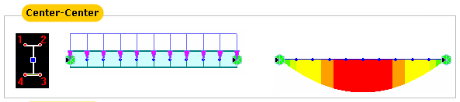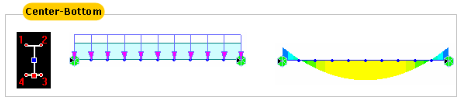User Offset Reference : When entering the offset distance for the section as the "User" type, you can specify the reference position that serves as the basis.

Centroid : Apply the offset distance entered based on the centroid of the section.

Extreme Fiber(s) : Apply the offset distance entered based on the position specified in "Offset" (Left/Right, Top/Bottom).When generating section data for Cold Formed Channels, precise section data is created by employing a precise calculation formula that takes into account the entire cross-section, including shear deformations.When specifying the User type, the offset distance and direction are always entered based on the centroid, regardless of the Center option (Centroid, Center of Section). For example, if you specify "Offset: Left-Center" and "Center Loc.: Center of Section" and enter a horizontal offset of "0.5" as User type, the offset position will be located "0.5" units to the left of the centroid.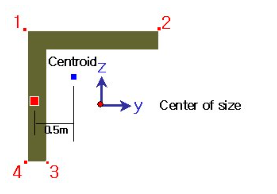Display Offset Point : Display the offset position entered in the Change Offset dialog in the illustration of the Section Data dialog.

### Section Property

Clickto display the section property data. The section property data table is either calculated from the main dimensions or obtained from the DB depending on the method of data entry.

Area : Cross sectional area

Asy : Effective Shear Area for shear force in the element's local y-direction

It becomes inactive when Shear Deformation is not considered.

Asz : Effective Shear Area for shear force in the element's local z-direction

It becomes inactive when Shear Deformation is not considered.

Ixx : Torsional constant about the element's local x-axis

Iyy : Moment of Inertia about the element's local y-direction

Izz : Moment of Inertia about the element's local z-direction

Cyp : Distance from the section's neutral axis to the extreme fiber of the element in the local (+)y-direction

Cym : Distance from the section's neutral axis to the extreme fiber of the element in the local (-)y-direction

Czp : Distance from the section's neutral axis to the extreme fiber of the element in the local (+)z-direction

Czm : Distance from the section's neutral axis to the extreme fiber of the element in the local (-)z-direction

Zyy : Plastic Section Modulus about the element local y-direction

Zzz : Plastic Section Modulus about the element local z-direction

Qyb : Shear Coefficient for the shear force applied in the element's local z-direction

Qzb : Shear Coefficient for the shear force applied in the element's local y-direction

Peri : O : Total perimeter of the section

Peri : I : Inside perimeter length of a hollow section

y1, z1 : Distance from the section's neutral axis to the Location 1 (used for computing combined stress)

y2, z2 : Distance from the section's neutral axis to the Location 1 (used for computing combined stress)

y3, z3 : Distance from the section's neutral axis to the Location 3 (used for computing combined stress)

y4, z4 : Distance from the section's neutral axis to the Location 4 (used for computing combined stress)All the above section property data except for Area and Peri are required for beam elements.The shear deformations are neglected if the effective shear areas are not specified. Cyp, Cym, Czp and Czm are used to calculate the bending stresses. Qyb and Qzb are used to calculate the shear stresses. Peri is used to calculate the Painting Area.Zyy and Zzz are used to calculate the strength for pushover analysis when Value Type Steel Section has been assigned Design > Pushover Analysis > Define Hinge Properties. In the case of the Ultimate condition, Pc (compressive), Pt (tensile), and M0 (bending strength at P=0, calculated as Fy×Zyy, Fy×Zzz) are used to generate the PM-Curve.Element Stiffness data

Area : Cross Sectional Area

Area : Cross Sectional Area

The cross-sectional area of a member is used to compute axial stiffness and stress when the member is subjected to a compression or tension force. Figure 1 illustrates the calculation procedure.

Cross-sectional areas could be reduced due to member openings and bolt or rivet holes for connections. midas Civil does not consider such reductions. Therefore, if necessary, the user is required to modify the values using the option 2 above and his/her judgment.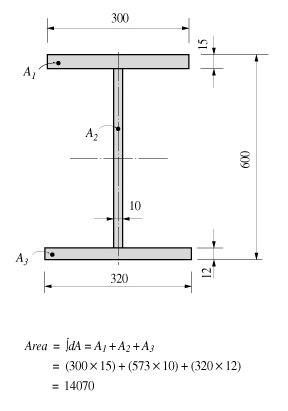<Figure 1 > Example of cross-sectional area calculation

Asy, Asz : Effective Shear Area

Asy, Asz : Effective Shear Area

The effective shear areas of a member are used to formulate the shear stiffness in the y- and z-axis directions of the cross-section. If the effective shear areas are omitted, the shear deformations in the corresponding directions are neglected.

When calculating section properties internally or when they are inputted from a database, the corresponding shear stiffness component is automatically considered, and the calculation method is as shown in Figure 2.

Asy : Effective shear area in the ECS y-axis direction

Asz : Effective shear area in the ECS z-axis direction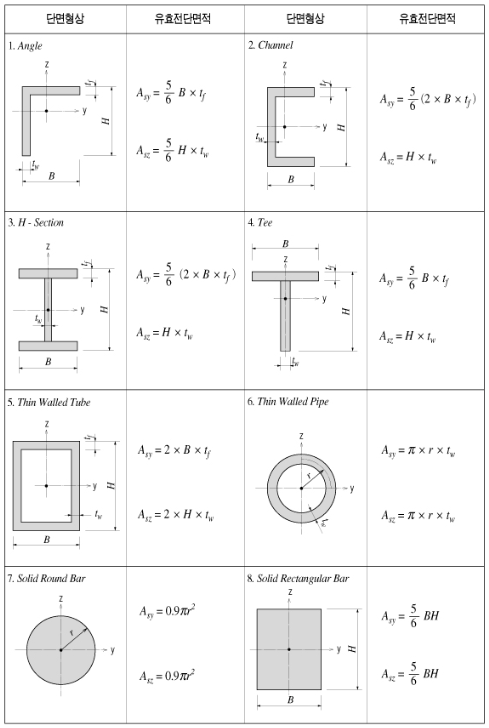<Figure 2> Effective shear area in the ECS z-axis direction

Ixx: Torsional Constant

Ixx: Torsional Constant

Torsional resistance refers to the stiffness resisting torsional moments. It is expressed as:

<Eq. 1>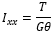where, Ixx : Torsional Constant

T : Torsional Moment or Torque

G : Shear Modulus of Elasticity

θ : Angle of Twist

The torsional stiffness expressed in Eq. 1 must not be confused with the polar moment of inertia that determines the torsional shear stresses.

However, they are identical to one another in the cases of circular or thick cylindrical sections.

No general equation exists to satisfactorily calculate the torsional resistance applicable for all section types. The calculation methods widely vary for open and closed sections and thin and thick thickness sections.

No general equation exists to satisfactorily calculate the torsional resistance applicable for all section types. The calculation methods widely vary for open and closed sections and thin and thick thickness sections.

<Eq. 2>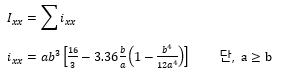where, ixx : Torsional resistance of a (rectangular) sub-section

2a : Length of the longer side of a sub-section

2b : Length of the shorter side of a sub-section

Figure 3 illustrates the equation for calculating the torsional resistance of a thin walled, tube-shaped, closed section.

<Eq. 3>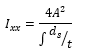where, Am : Area enclosed by the mid-line of the tube

ds : Infinitesimal length of thickness centerline at a given point

t : Thickness of tube at a given point

For those sections such as bridge box girders, which retain the form of thick walled tubes, the torsional stiffness can be obtained by combining the above two equations, Eq. 1 and Eq. 3.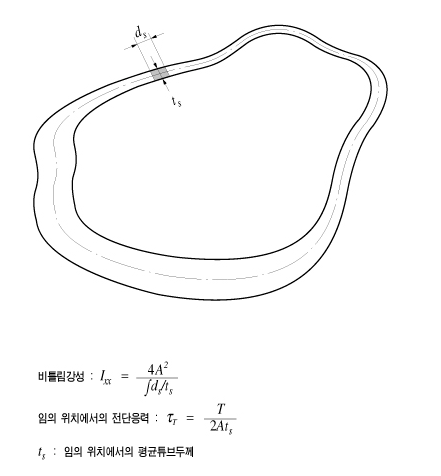<Figure 3> Torsional resistance of a thin walled, tube-shaped, closed section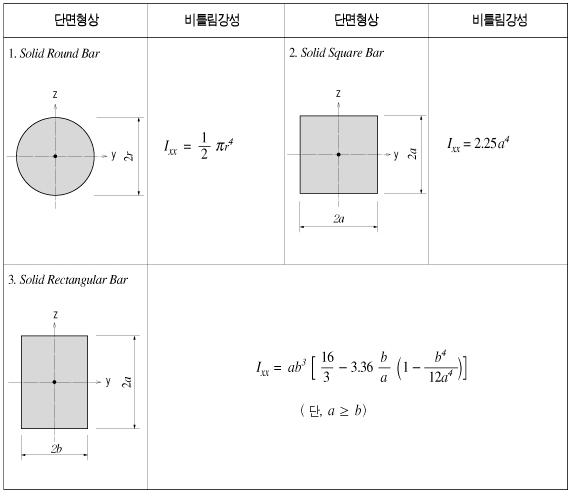<Figure 4> Torsional resistance of solid sections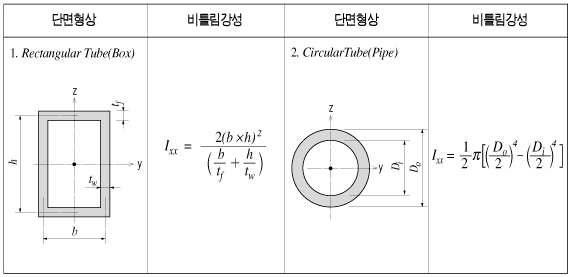<Figure 5> Torsional resistance of thin walled, closed sections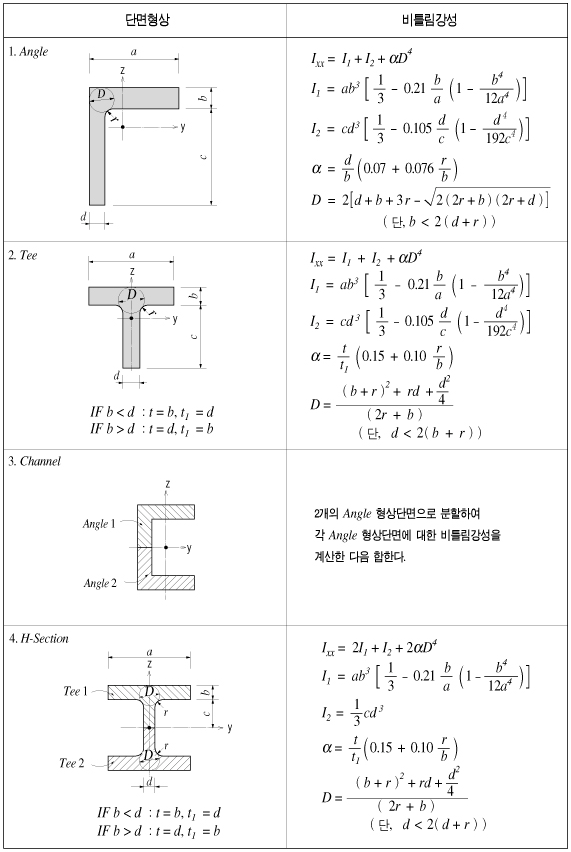<Figure 6> Torsional resistance of thick walled, open sections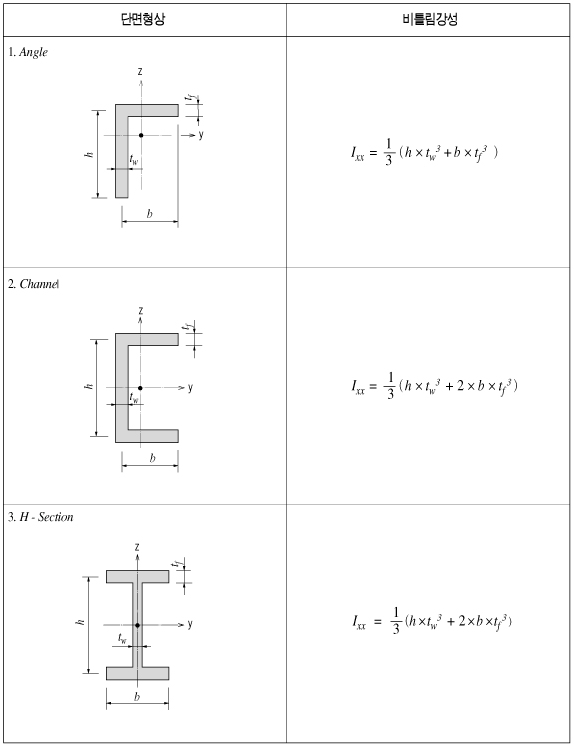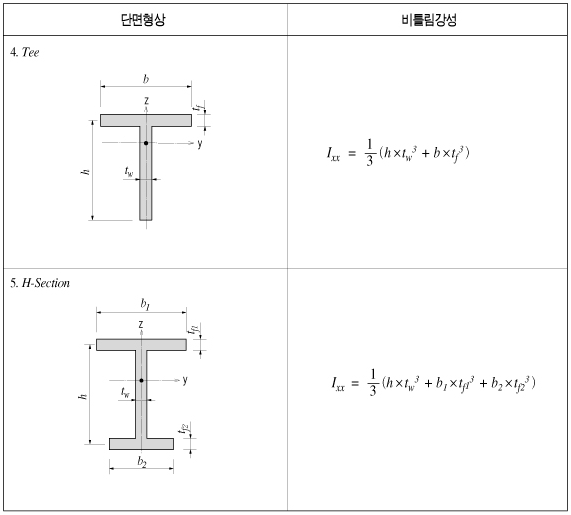<Figure 7> Torsional resistance of thin walled, open sections

In practice, combined sections often exist. A combined built-up section may include both closed and open sections. In such a case, the stiffness calculation is performed for each part, and their torsional stiffnesses are summed to establish the total stiffness for the built-up section.

For example, a double I-section shown in Figure 8(a) consists of a closed section in the middle and two open sections, one on each side.

The torsional resistance of the closed section (hatched part)

<Eq. 4>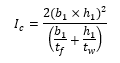The torsional resistance of the open sections (unhatched parts)

<Eq. 5>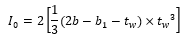The total resistance of the built-up sections

<Eq. 6>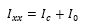Figure 8(b) shows a built-up section made up of an I-shaped section reinforced with two web plates, forming two closed sections. In this case, the torsional resistance for the section is computed as follows:

If the torsional resistance contributed by the flange tips is negligible relative to the total section, the torsional property may be calculated solely on the basis of the outer closed section (hatched section) as expressed in Eq. 7.

<Eq. 7>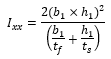If the torsional resistance of the open sections is too large to ignore, then it should be included in the total resistance.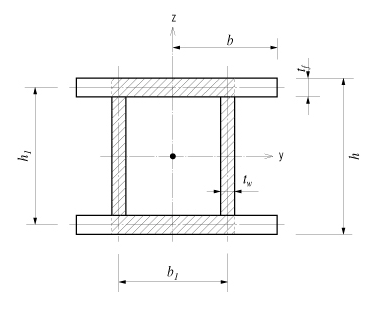(a) Section consisted of closed and open sections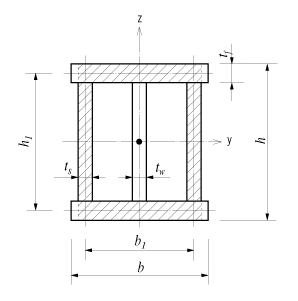(b) Section consisted of closed and open sections

<Figure 8> Torsional resistance of built-up sections

Iyy, Izz: Area Moment of Inertia

Iyy, Izz: Area Moment of Inertia

The area moment of inertia is used to compute the flexural stiffness resisting bending moments. It is calculated relative to the centroid of the section.

Moment of inertia about the y-axis in the element coordinate system

<Eq. 1>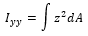Moment of inertia about the z-axis in the element coordinate system

<Eq. 2>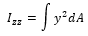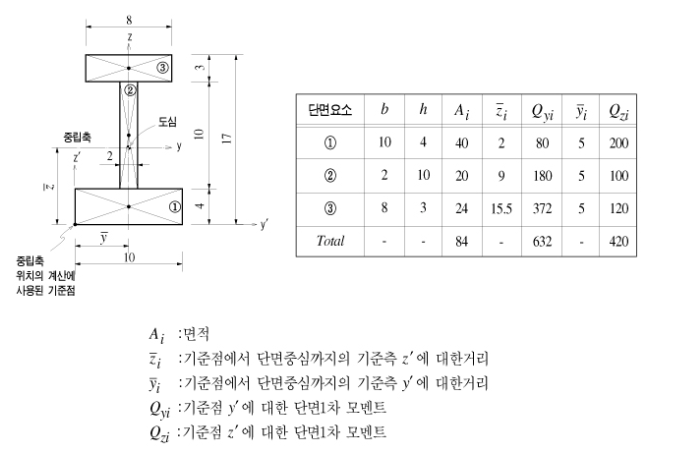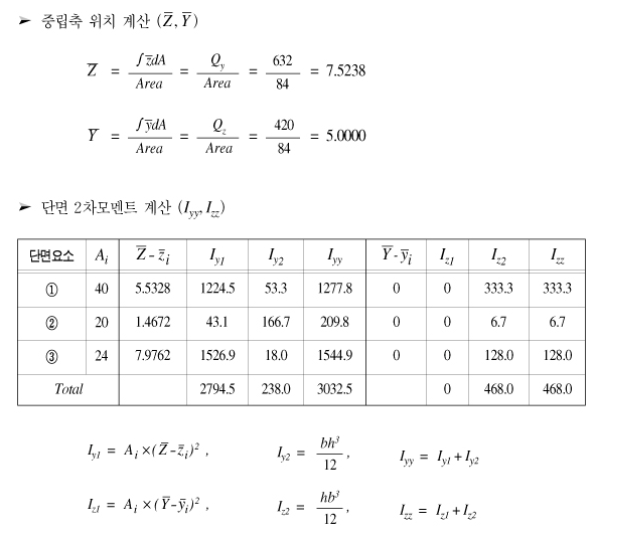<Figure 9> Example of the calculation for moment of inertia

Iyz: Area Product Moment of Inertia

Iyz: Area Product Moment of Inertia

The area product moment of inertia is used to compute stresses for non-symmetrical sections, which is defined as follows:

<Eq. 1>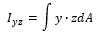Sections that have at least one axis of symmetry produce Iyz=0. Typical symmetrical sections include I, pipe, box, channel and tee shapes, which are symmetrical about at least one of their local axes, y and z.

However, for non-symmetrical sections such as angle shaped sections, where Iyz≠0, the area product moment of inertia should be considered for obtaining stress components.

The area product moment of inertia for an angle is calculated as shown in Figure 10.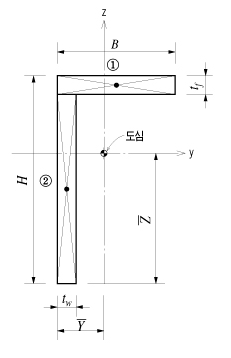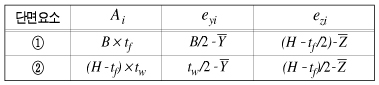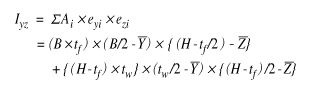<Figure 10> Area product moment of inertia for an angle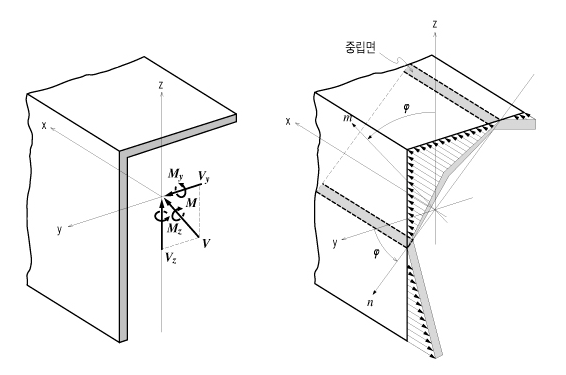<Figure 11> Bending stress distribution of a non-symmetrical section

The neutral axis represents an axis along which bending stress is 0 (zero). As illustrated in the right-hand side of Figure 11, the n-axis represents the neutral axis, to which the m-axis is perpendicular.

Since the bending stress is zero at the neutral axis, the direction of the neutral axis can be obtained from the relation defined as:

In the neutral axis, the bending stress due to the bending moment is '0', allowing us to determine the direction of the neutral axis using the following relationship.

<Eq. 2>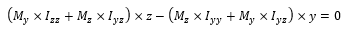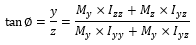The general equation applied to calculate the bending stress in a section due to bending moment is as follows.

<Eq. 3>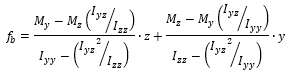In the case of an I shaped section, Iyz=0, hence the equation can be simplified as:

<Eq. 4>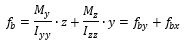where, Iyy : Area moment of inertia about the ECS y-axis

Izz : Area moment of inertia about the ECS y-axis

Iyz : Area product moment of inertia

y :  Distance from the neutral axis to the location of bending stress calculation in the ECS y-axis direction

z : Distance from the neutral axis to the location of bending stress calculation in the ECS z-axis direction

My : Bending moment about the ECS y-axis

Mz : Bending moment about the ECS z-axis

The general expressions for calculating shear stresses in the ECS y and z-axes are:

<Eq. 5>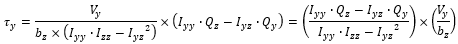<Eq. 6>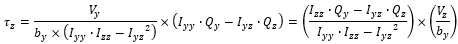where, Vy : Shear force in the ECS y-axis direction

Vz : Shear force in the ECS z-axis direction

Qy : First moment of area about the ECS y-axis

Qz : First moment of area about the ECS z-axis

by : Thickness of the section at which a shear stress is calculated, in the direction normal to the ECS z-axis

bz : Thickness of the section at which a shear stress is calculated, in the direction normal to the ECS y-axis

Qy, Qz: First Moment of Area

Qy, Qz: First Moment of Area

The first moment of area is used to compute the shear stress at a particular point on a section. It is defined as follows:

<Eq. 1>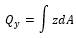<Eq. 2>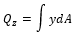When a section is symmetrical about at least one of the y and z-axis, the shear stresses at a particular point are:

<Eq. 3>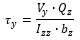<Eq. 4>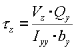where, Vy : Shear force acting in the ECS y-axis direction

Vz : Shear force acting in the ECS z-axis direction

Iyy : Area moment of inertia about the ECS y-axis

Izz : Area moment of inertia about the ECS z-axis

by : Thickness of the section at the point of shear stress calculation in the ECS y-axis direction

bz : Thickness of the section at the point of shear stress calculation in the ECS z-axis direction

Qyb, Qzb: Shear Factors of Shear Stress due to Bending

Qyb, Qzb: Shear Factors of Shear Stress due to Bending

The shear factor is used to compute the shear stress at a particular point on a section, which is obtained by dividing the first moment of area by the thickness of the section.

<Eq. 1>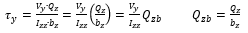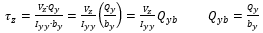<Eq. 2>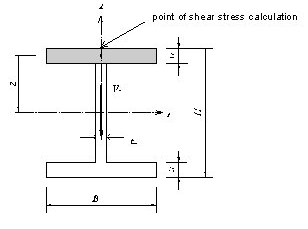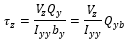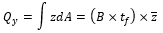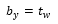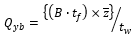<Figure 12> Example of calculating a shear factor

Stiffness of Composite Sections

Stiffness of Composite Sections

midas Civil calculates the stiffness for a full composite action of structural steel and reinforced concrete. Reinforcing bars are presumed to be included in the concrete section. The composite action is transformed into equivalent section properties.

The program uses the elastic moduli of the steel (Es) and concrete (Ec) defined in the SSRC79 (Structural Stability Research Council, 1979, USA) for calculating the equivalent section properties. In addition, the Ec value is decreased by 20% in accordance with the EUROCODE 4.

Equivalent cross-sectional area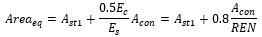Equivalent effective shear area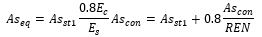Equivalent area moment of inertia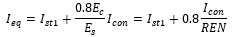where, Ast1 : Area of structural steel

Acon : Area of structural steel

Asst1 : Effective shear area of structural steel

Ascon : Effective shear area of concrete

Ist1 : Area moment of inertia of structural steel

Icon : Area moment of inertia of concrete

REN : Modular ratio (elasticity modular ratio of the structural steel to the concrete, Es/Ec)

Equivalent torsional coefficient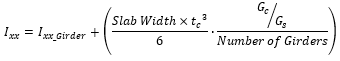Determining the positions of y1~4, z1~4 of a section imported from SPC

Bending stress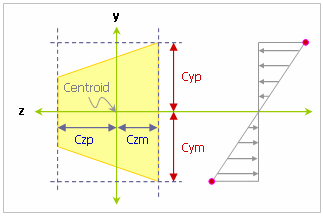Combined Normal Stress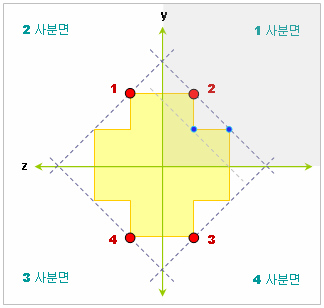1. Divide the section into four quadrants.

2. Draw a straight line passing through each point that forms the cross-section with a slope of "1" or "-1."

3. Find the straight line with the largest absolute value of the y-intercept among multiple lines.

4. Determine the points of contact with the respective line as the locations for stress calculation.

If there are multiple points with the same magnitude of the y-intercept, the point with the larger absolute value of the y-coordinate is chosen as the location for stress calculation.

0